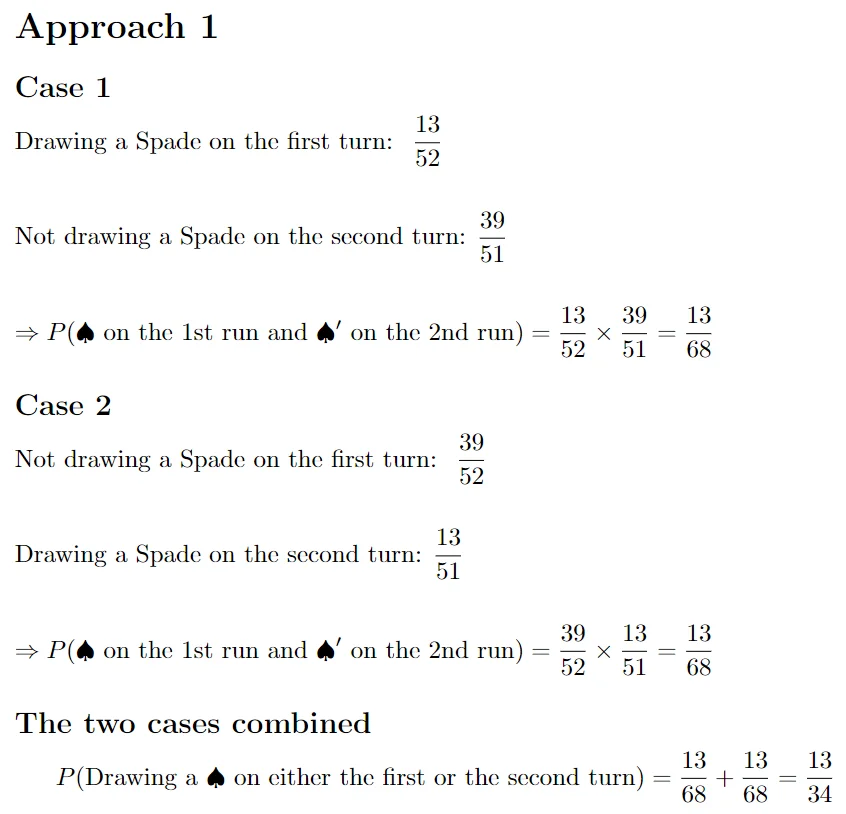04 May 2023

Posted on:

05 Jan 2023

0

# Lecture Homework Solution

Find the probability of getting a spade on either the first turn or the second turn

P(A) = 13/52=0.25
P(B) = 13/52=0.25
P(A|B) = 12/51 ~ 0.2353

P(AUB) = P(A) + P(B) - P(A∩B)  [Additive Law]
= P(A) + P(B) - P(A|B) * P(B)     [Multiplication Law]
= 0.25 + 0.25 - 0.2353*0.25
= 0.5 - 0.0588
= 0.4412

Posted on:

06 Jan 2023

2

Hey Eslam,

Thank you for reaching out!

Note that the task asks for the probability of drawing a spade on either the first turn or the second turn. This means we need to study the following 2 cases:

Case 1: Drawing a spade on the first turn and not drawing a spade on the second turn.
Case 2: Not drawing a spade on the first turn and drawing a spade on the second turn.

Find the probabilities of both of these cases, sum them up, and you'll find the probability of drawing either on the first, or on the second turn.

Another approach to solve the same problem is to instead consider the following 2 cases:
Case 1: Drawing a spade on both the first and the second turn.
Case 2: Drawing a spade on neither the first, nor the second turn.

Find the probabilities of both of these cases, sum them up, find their complement, and you should obtain the same result as the first approach.

Hope this helps!

Kind regards,
365 Hristina

Posted on:

04 Apr 2023

0

hI TEAM,

can u please provide the answer for above to help understand the right way?

Thx

Anu

Posted on:

05 Apr 2023

2

Thank you for reaching out!

In the following screenshot, I've outlined the solution to the first approach suggested in the previous answer. You can solve for the second approach in a similar fashion and ensure that the result is the same.Kind regards,

365 Hristina

Posted on:

04 May 2023

1

Hi there!
Please continue the solution as in the lecture to see the full picture, and why and how we can use the multiplication rule.

Thanks